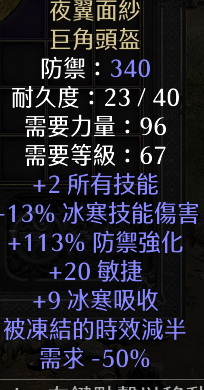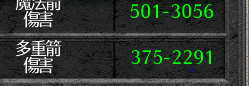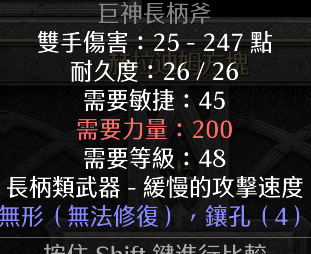//没登陆

 本帖最后由 风apex 于 2021-10-29 18:37 编辑没了 真的就这么点东西 底材？不存在的 符文 没看到哇
33=0      格里芬=1 破隐=0 年纪=0 次元=0
32=0     11.14 无限   12.12谜团
31=0
30=2
29=1
28=4 （11.5 第8次超市 11.27 3C）
27=3
26=2
25=10
24=5
23=8
22=5
 弓的mf。。。不建议去，地穴400mf起步

 敢穿400MF 的弓马 怕是一背包锐利了吧

33=0      格里芬=1 破隐=0 年纪=0 次元=0
32=0     11.14 无限   12.12谜团
31=0
30=2
29=1
28=4 （11.5 第8次超市 11.27 3C）
27=3
26=2
25=10
24=5
23=8
22=5
 CORSTI 发表于 2021-10-29 17:34 弓的mf。。。不建议去，地穴400mf起步 你在说什么呢，地穴要mf？

 弓就适合，直接a4射穿，去地穴犄角旮旯，低级怪沉沦魔的nodrop概率应该70%以上，伤到家，啥都不掉

 弓马1pp还是去超市吧

 我觉得三小时能出这个不错了

 我只是来随缘摸符文的  弓马只是信仰

33=0      格里芬=1 破隐=0 年纪=0 次元=0
32=0     11.14 无限   12.12谜团
31=0
30=2
29=1
28=4 （11.5 第8次超市 11.27 3C）
27=3
26=2
25=10
24=5
23=8
22=5这面板去A4 太慢了

33=0      格里芬=1 破隐=0 年纪=0 次元=0
32=0     11.14 无限   12.12谜团
31=0
30=2
29=1
28=4 （11.5 第8次超市 11.27 3C）
27=3
26=2
25=10
24=5
23=8
22=5
 起个冰冻弓吧。。。。。意思明摆着快4个小时了又一个这个

33=0      格里芬=1 破隐=0 年纪=0 次元=0
32=0     11.14 无限   12.12谜团
31=0
30=2
29=1
28=4 （11.5 第8次超市 11.27 3C）
27=3
26=2
25=10
24=5
23=8
22=5
 风apex 发表于 2021-10-29 17:40 这面板去A4 太慢了 你需要刚毅

 下午 QS 打瘸子出了个30 还没时间去找人换 28

33=0      格里芬=1 破隐=0 年纪=0 次元=0
32=0     11.14 无限   12.12谜团
31=0
30=2
29=1
28=4 （11.5 第8次超市 11.27 3C）
27=3
26=2
25=10
24=5
23=8
22=5
 换了 28 也想先给米爹做一件

33=0      格里芬=1 破隐=0 年纪=0 次元=0
32=0     11.14 无限   12.12谜团
31=0
30=2
29=1
28=4 （11.5 第8次超市 11.27 3C）
27=3
26=2
25=10
24=5
23=8
22=5